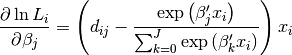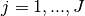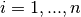# statsmodels.discrete.discrete_model.MNLogit.score_obs¶

MNLogit.score_obs(params)[source]

Jacobian matrix for multinomial logit model log-likelihood

Parameters: params : array The parameters of the multinomial logit model. jac : ndarray, (nobs, k_vars*(J-1)) The derivative of the loglikelihood for each observation evaluated at params .

Notesfor, for observationsIn the multinomial model the score vector is K x (J-1) but is returned as a flattened array. The Jacobian has the observations in rows and the flatteded array of derivatives in columns.